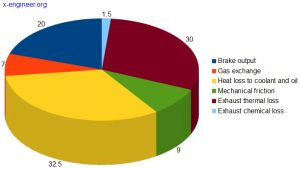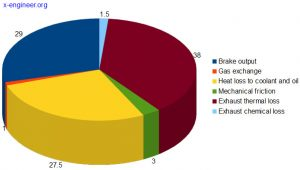# Mechanical efficiency and friction mean effective pressure (FMEP)

Internal combustion engines are known to have low efficiency. This is because most of the energy available from combustion is not converted into useful work, at the crankshaft, but lost (dissipated), mostly through heat transfer and friction.

For a typical engine, only a third of the combustion energy (at full load) ends up at the crankshaft (brake output). The situation is even worse at part load, where the efficiency of the engine is around 20%. In the table below you can see the distribution (in percentage) of energy losses of an internal combustion engine at part load and at full load.

 Energy share Part load Full load Brake output (useful energy) 20 % 29 % Gas exchange losses 7 % 1 % Heat losses to coolant and oil 32.5 % 27.5 % Mechanical friction losses 9 % 3 % Exhaust thermal losses 30 % 38 % Exhaust chemical losses 1.5 % 1.5 %

The numbers in the table are plotted in the pie charts below.Image: Engine losses at part load Credit: FEVImage: Engine losses at full load Credit: FEV

At idle speed, the brake output of the engine is 0 %, because all the combustion energy is used to maintain the engine speed. This means that, at idle speed, the energy losses of the engine is 100%.

### Friction mean effective pressure (FMEP)

The lost mechanical work is regarded as “friction” work. It has the generic name “friction” but it contains all the engine losses: pumping losses, actual friction (mechanical rubbing) losses and auxiliary devices (accessory) losses.

Through the combustion process we get a theoretical amount of work which can be used for propulsion, called indicated work Wi. Subtracting the friction losses work Wf, we’ll end up with an effective work We, which can be used for propulsion.

$W_e = W_i – W_f \tag{1}$

The “friction” losses work is made up from:

• pumping work Wp
• rubbing friction work Wr
• auxiliary devices work Wa
$W_f = W_p + W_r + W_a \tag{2}$

We can also use normalised parameters, like mean effective pressure (MEP), to define “friction” losses. The brake mean effective pressure (BMEP) is what we have at the crankshaft. BMEP is the difference between indicated mean effective pressure (IMEP) and “friction” mean effective pressure (FMEP).

$BMEP = IMEP – FMEP \tag{3}$

where the “friction” mean effective pressure (FMEP) is the sum of pumping mean effective pressure (PMEP), mechanical rubbing mean effective pressure (RMEP) and auxiliary mean effective pressure (AMEP)

$FMEP = PMEP + RMEP + AMEP \tag{4}$

### Mechanical efficiency

The mechanical efficiency of the engine is the ratio between the brake mean effective pressure and the indicated mean effective pressure:

$\eta_m = \frac{BMEP}{IMEP} \tag{5}$

Replacing equation (3) in (5) gives:

$\eta_m = \frac{IMEP-FMEP}{IMEP}=1-\frac{FMEP}{IMEP} \tag{6}$

From equation (6) we can see that, the lower is the friction mean effective pressure (FMEP), the higher is the mechanical efficiency of the internal combustion engine. So, reducing the “friction” losses for a given engine, will reduce the fuel consumption and improve the power output.

Also, the heat losses of an internal combustion engine need to be dissipated by the cooling system and the lubrication system. Lowering the FMEP will reduce the component size in the cooling and lubrication system, which means further efficiency improvement.

Since the internal combustion engine is a complex machinery, with a multitude of components and systems, there are a lot of sources for the energy losses:

• mechanical rubbing losses: piston assembly, connecting rod, crankshaft, balance shaft, valve train system
• pumping losses: intake and exhaust
• auxiliary device losses: oil pump, fuel pump, water pump, alternator, AC compressor, etc.

The sum of all these losses are represented as friction mean effective pressure (FMEP). The losses are not constant through the engine speed range, but they are increasing with the engine speed.

The moving parts of the engine (piston assembly, connection rod, crankshaft and valve train) account for more than half of the FMEP. Between these components, half of the losses come from the piston assembly:

 Components Average values of the friction losses Piston assembly 49 % Crankshaft system 18 % Connecting rod system 16 % Valve train system 17 %

The friction losses are not constant, they depend on engine speed and temperature. The lower the temperature, the higher the oil viscosity, the higher the friction losses.

Because temperature plays a key role in the engine efficiency, the warm-up phase of the engine should be as short as possible and the temperature ramped up to nominal value as fast as possible.

### Friction mechanics

Friction occurs when two bodies are in contact with each other and have relative motion. All the moving parts of an internal combustion engine are lubricated (with oil or grease) so the friction is wet.

At a microscopic level, friction is caused by the asperities of the contact surfaces. Even if there is a layer of lubricant between the two surfaces, depending on the thickness of the lubricant layer, the asperities might come into contact, causing dry friction.

The friction force Ff [N] depends on the friction coefficient μ [-] and the normal force N [N]. The normal force is the force perpendicular to the friction surface.

$F_f = \mu \cdot N \tag{7}$

To minimize the friction force we either lower the friction coefficient or the normal force. The coefficient of friction can be expressed as:

$\mu = \alpha \cdot \mu_s + (1 – \alpha) \cdot \mu_L \tag{8}$

where:

μs [-] – is the dry friction coefficient (metal to metal)
μL [-] – is the hydrodynamic (wet) friction coefficient
α [-] – is the surface contact constant, varying between 1 and 0

Depending on the value of the surface contact constant α, the friction can be:

Case I. α = 1

$\mu = \mu_s$

In this case we have boundary friction, in which the surfaces asperities are longer than the height of the lubricant layer. The friction is mainly metal to metal (dry friction).

Case II. α = 0

$\mu = \mu_L$

In this case we have hydrodynamic friction, in which the surfaces asperities are shorter than the lubricant layer. There is not metal to metal contact between surfaces, the friction taking place only between the layers of the lubricant.

Case II. α between 0 and 1

In this case we have a mixed friction between the two surfaces. The height of the asperities are nearly equal with the lubricant thickness so there is a mix between hydrodynamic friction and boundary (dry) friction.

In the Stribeck diagram below we can see the shape of the friction coefficient, for each of the above mentioned cases. The diagram describes the variation of the friction coefficient between two liquid-lubricated surfaces as a function of a dimensionless lubrication parameter.

where:

h [mm] – is the height of the lubricant layer
Ra [mm] – is the height of the contact surface asperities
N [N] – normal force
v [m/s] – speed

In an internal combustion engine, especially after a long period of inactivity, at engine start, we have boundary friction between components. With the oil pump in action, the lubricant layer becomes thicker and the friction becomes hydrodynamic.

Taking into account the friction phenomena, we can write down several methods of improving the overall mechanical efficiency of the internal combustion engine:

• decrease the value of the static friction coefficient μs: this can be achieved by using materials with low friction coefficient on the piston skirt or the piston rings
• decrease the value of the hydrodynamic friction coefficient μL: by using low viscosity lubricants (oil) such as 0W-20 or even 0W-16
• optimisation of the lubrication system in order to minimise the time spent in mixed friction (transitory phase)
• optimisation of the thermal management of the engine in order to warm-up as fast as possible
• use electrical actuated pumps (oil, water) and compressors (AC)

### Engine friction model

In engineering, there is a need to approximate the friction mean effective pressure (FMEP) with an empirical equation. This is useful when the engineer needs to calculate the fuel efficiency of the engine on a given driving cycle.

The Chen-Flynn friction correlation model can be used to calculate the FMEP of an engine. According to this model, the FMEP [bar] is function of the cylinder maximum pressure pmax [bar] and an engine speed factor Sf [rad·m/s].

$FMEP = A + B \cdot p_{max} + C \cdot S_f + D \cdot S_f^2 \tag{9}$

where:

A [bar] – constant, which accounts for the auxiliary losses and other invariable factors
B [-] – constant, which accounts for the load effect of the engine (in-cylinder pressure)
C [bar·s/m], D [bar·s2/m2] – constants, which accounts for the effect of the engine speed (as a quadratic law)

The speed factor is calculated as:

$S_f = \frac{\omega \cdot S}{2} \tag{10}$

where:

S [m] – piston stroke

Engine speed is converted from [rpm] to [rad/s] with the formula:

$\omega = N \cdot \frac{\pi}{30} \tag{11}$

where N [rpm] is the engine speed.

Example. Calculate and plot the friction mean effective pressure for a 2 litre, in-line, 4 cylinder gasoline engine, with the following friction correlation parameters:

$\begin{split} p_{max} &= 12 \text{ bar}\\ N &= 1000 \text{ … } 6000 \text{ rpm}\\ S &= 82 \cdot 10^{-3} \text{ m}\\ A &= 0.3 \text{ bar}\\ B &= 0.006 \\ C &= 0.05 \text{ } \frac{\text{bar} \cdot \text{s}}{\text{m}}\\ D &= 85 \cdot 10^{-5} \text{ } \frac{\text{bar} \cdot \text{s}^2}{\text{m}^2} \end{split}$

The easiest way to calculate FMEP is to write a Scilab script with the equations (9), (10), (11) and parameters:

pmax = 12; // bar
N = [1000:100:6000]; // rpm
S = 82e-3;
A = 0.3; // bar
B = 0.006;
C = 0.05; // bar*s/m
D = 85e-5; // bar*s2/m2

for i=1:length(w)
Sf(i) = S/2 * w(i);
FMEP(i) = A + B*pmax + C * Sf(i) + D * Sf(i)^2;
end

plot(N,FMEP,'LineWidth',3);
ha=gca();
ha.tight_limits = ["on","on","on"];
ha.data_bounds=[0,0;max(N)+1000,2.5];
xgrid();
xlabel('Engine speed [rpm]','FontSize',3);
ylabel('FMEP [bar]','FontSize',3);
title('x-engineer.org','FontSize',2);


Running the script will output the following graphical window:

There are several methods of measuring the FMEP on the actual engine. Two of these methods are the floating liner method and the instantaneous IMEP method. The floating liner method has the advantage of calculating friction directly, but it involves major engine modification which makes it expensive. On the other hand, instantaneous IMEP method calculates friction indirectly by subtracting two big quantities to obtain a small value.

Don’t forget to Like, Share and Subscribe!